# Methods and formulas for Variables Acceptance Sampling (Accept/Reject Lot)

Minitab calculates an accept or reject decision based on your measurements from sampled items and the criteria (sample size and critical distance) of your variables acceptance sampling plan.

First Minitab calculates the mean and standard deviation from your data (if you have not specified a historical standard deviation):

Mean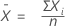Standard deviation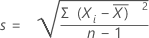Acceptance criteria for specification limits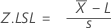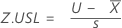Maximum Standard Deviation (MSD)
If you have 2 specification limits and the standard deviation is unknown, then the MSD plays a role in accepting or rejecting the lot. For the calculation of MSD, go to Methods and formulas for Variables Acceptance Sampling (Create/Compare).
###### Note

Minitab uses σ instead of s in the Z calculations when you provide a historical standard deviation.

Single specification limit
The corresponding Z-value is calculated. If lower specification only, accept the lot if Z.LSL ≥ k; otherwise reject the lot. If upper specification only, accept the lot if Z.USL ≥ k; otherwise reject the lot.
Double specification limits and known standard deviation
The Z-values for each specification are calculated. Accept the lot if Z.LSL ≥ k and Z.USL ≥ k; otherwise reject the lot.
Double specification limits and unknown standard deviation
The Z-values for each specification and the MSD are calculated. Accept the lot if s ≤ MSD, Z.LSL ≥ k and Z.USL ≥ k; otherwise reject the lot.

## Notation

TermDescription
Ximeasurement data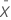mean
sestimated standard deviation
σknown standard deviation
nsample size
kcritical distance
Llower specification limit
Uupper specification limit